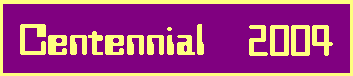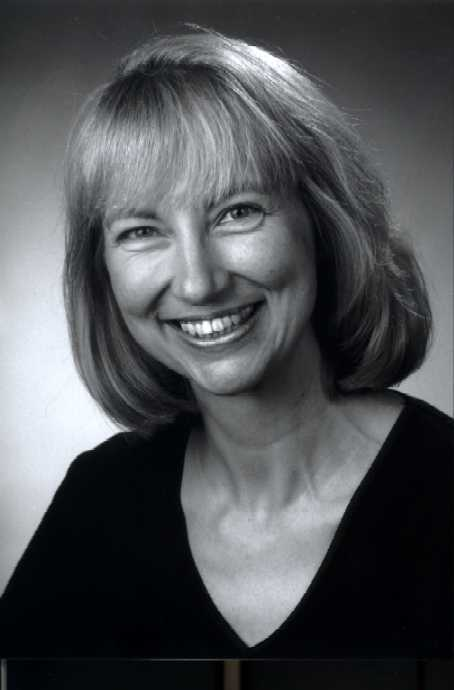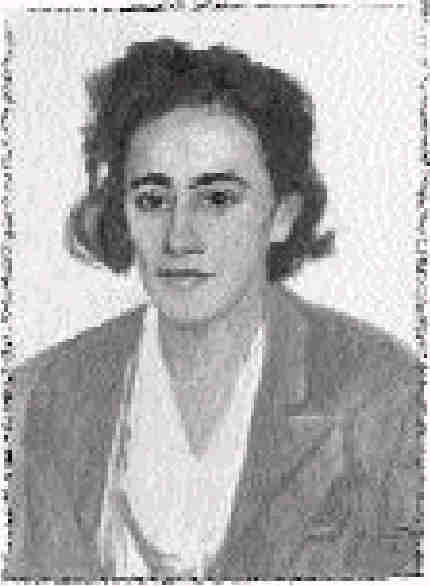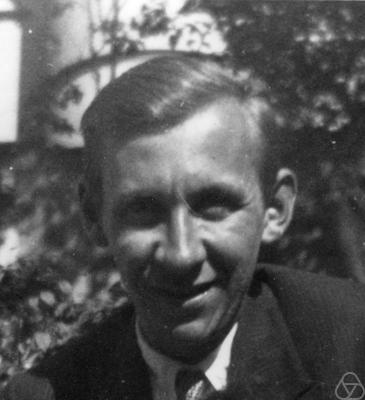# 2-Stage Metabelian 3-Groups

## 1. Classical scenario:

### The Galois group of the 2nd Hilbert 3-class fieldThe original purpose of Brigitte Nebelung's thesis  , written in 1989 under the supervision of Wolfram Jehne at Cologne, was to gain a thorough overview of all 2-stage metabelian 3-groups G with commutator factor group G/G' of type (3,3) and to apply the results to the following problems which were first posed by Arnold Scholz and Olga Taussky-Todd in 1933 (inspired by the Furtwängler / Artin proof of the principal ideal theorem) for imaginary quadratic fields K  but arise for any algebraic number field K with 3-class group Syl3C(K) of type (3,3):

1. to determine the Galois group G(K2|K) of the 2nd Hilbert 3-class field K2 of K over K,
2. to find the structure of the 3-class group Syl3C(K1) of the 1st Hilbert 3-class field K1 of K.

 K2 | K1 | K
 G(K2|K2) = 1 | G' = G(K2|K1) = Syl3C(K1) ---- | G/G' = G(K1|K) = Syl3C(K) = (3,3) G = G(K2|K) ----

This application is due to class field theory, since K1 is the maximal abelian unramified 3-extension of K and thus the subgroup U = G(K2|K1) of G = G(K2|K) with factor group G/U = G(K1|K) = Syl3C(K) = (3,3) must be the minimal subgroup of G with abelian factor group, i. e., must coincide with the commutator subgroup G' of G. Further, G is a 2-stage metabelian 3-group, since G' = G(K2|K1) = Syl3C(K1) is abelian, i.e., G'' = 1.

Warning:
Although taking G = G(Kn|K), for some integer n >= 3, similarly yields G' = G(Kn|K1) and G/G' = (3,3), the Galois group G = G(Kn|K) of the nth Hilbert 3-class field Kn of K over K is not a 2-stage metabelian 3-group any longer, in general, since G'' = G(Kn|K2) != 1, if 3 divides the class number of K2.

Top recent applications of the present theory have been developed in the following two articles:
A. The Galois group of the 2nd Hilbert 3-class field over a real quadratic field
B. The Galois group of the 2nd Hilbert 3-class field over a complex quadratic field
 References:  Arnold Scholz und Olga Taussky, Die Hauptideale der kubischen Klassenkörper imaginär quadratischer Zahlkörper, J. reine angew. Math.171 (1934), 19 - 41  Franz-Peter Heider und Bodo Schmithals, Zur Kapitulation der Idealklassen in unverzweigten primzyklischen Erweiterungen, J. reine angew. Math. 336 (1982), 1 - 25  James R. Brink and Robert Gold, Class field towers of imaginary quadratic fields, manuscripta math. 57 (1987), 425 - 450  Brigitte Nebelung, Klassifikation metabelscher 3-Gruppen mit Faktorkommutatorgruppe vom Typ (3,3) und Anwendung auf das Kapitulationsproblem, Inauguraldissertation, Köln, 1989  Daniel C. Mayer, Principalization in complex S3-fields, Congressus Numerantium 80 (1991), 73 - 87  Daniel C. Mayer, List of discriminants dL<200000 of totally real cubic fields L, arranged according to their multiplicities m and conductors f, 1991, Dept. of Comp. Sci., Univ. of Manitoba  Daniel C. Mayer, Principalization in Unramified Cyclic Cubic Extensions of all Quadratic Fields with Discriminant -50000 < d < 0 and 3-Class Group of Type (3,3), Univ. Graz, Computer Centre, 2003

 <| Navigation Center <| <| Back to Algebra <|